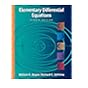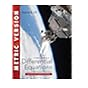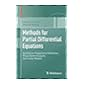Normal view

A First course in differential equations with modeling applications / Dennis G. Zill.

Material type:TextLanguage: English Publication details: 2018. Edition: Eleventh edition; Metric editionDescription: x, 389 pages : illustrations ; 28 cmContent type: text Media type: unmediated Carrier type: volumeISBN: 9781337556644Other title: First course in differential equations with modeling applicationsSubject(s): Differential equations -- TextbooksLOC classification: QA372 | Z6 2018
Contents:
Introduction to differential equations -- First-order differential equations -- Modeling with first-order differential equations -- Higher-order differential equations -- Modelling with higher-order differential equations -- Series solutions of linear equations -- The Laplace transform -- Systems of linear first-order differential equations -- Numerical solutions of ordinary differential equations -- Appendices -- Answers to selected odd-numbered problems -- Index.
Summary: Straightforward and easy to read, A FIRST COURSE IN DIFFERENTIAL EQUATIONS WITH MODELING APPLICATIONS, 11E, INTERNATIONAL METRIC EDITION, gives you a thorough overview of the topics typically taught in a first course in differential equations. Your study of differential equations and its applications will be supported by a bounty of pedagogical aids, including an abundance of examples, explanations, "Remarks" boxes, definitions, and more.
List(s) this item appears in: New Print Books 2021
Star ratingsAverage rating: 0.0 (0 votes)
Holdings
Item type Current library Collection Call number Materials specified Status Notes Date due BarcodeBooks Ladislao N. Diwa Memorial Library
Reserve Section
Non-fiction RUS QA372 Z6 2018 (Browse shelf(Opens below)) Room use only 76938 00078023
Browsing Ladislao N. Diwa Memorial Library shelves, Shelving location: Reserve Section, Collection: Non-fiction Close shelf browser (Hides shelf browser)RUS QA371 B69 2005 Elementary differential equations / RUS QA372 Or2 2016 Ordinary differential equations / RUS QA372 Or2 2017 Ordinary differential equations / RUS QA372 Z6 2018 A First course in differential equations with modeling applications / RUS QA377 Eb3 2018 Methods for partial differential equations : qualitative properties of solutions, phase space analysis, semilinear models / RUS QA43 B61 2004 College mathematics II / RUS QA453 H38 2005 Experiencing geometry : euclidean and non-euclidean with history /

"Metric version."--Front cover.

Includes index.

Introduction to differential equations -- First-order differential equations -- Modeling with first-order differential equations -- Higher-order differential equations -- Modelling with higher-order differential equations -- Series solutions of linear equations -- The Laplace transform -- Systems of linear first-order differential equations -- Numerical solutions of ordinary differential equations -- Appendices -- Answers to selected odd-numbered problems -- Index.

Straightforward and easy to read, A FIRST COURSE IN DIFFERENTIAL EQUATIONS WITH MODELING APPLICATIONS, 11E, INTERNATIONAL METRIC EDITION, gives you a thorough overview of the topics typically taught in a first course in differential equations. Your study of differential equations and its applications will be supported by a bounty of pedagogical aids, including an abundance of examples, explanations, "Remarks" boxes, definitions, and more.

Fund 164 Belview Co., Inc. Purchased 04/25/2019 76938 NEJ PHP 5,880.00 2019-84-299 2019-1-0256